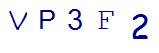# Be the Hero: How to Help Solve the Most Common 6th Grade Math ProblemsLast Updated on August 31, 2021 by Thinkster

Do your child’s 6th grade math problems seem incredibly hard? If you are looking to impress your child, and help him with his homework, consider these common problems. By learning how to solve them, you can help take your child’s excitement about math concepts to the next level.

## Ratio Word Problems

Ratios are one area where math meets the real world, and sometimes kids struggle with this particular aspect of math class. Ratio compares two numbers and is one of the common 6th grade math problems that can stump students.

Consider this problem: “In history class, the boy to girl ratio is 5 to 8. If there are a total of 65 students, how many boys are in the class?”

This problem is telling you that for every 5 boys, the class has 8 girls.

To solve, you need to find the ratio of boys to total students. Write this as a fraction, boys/total students.

Now, you need to create another ratio (fraction) using the information you have. Since boys are the numerator of the fraction you already created, then use the 5 as the numerator of the second fraction. Now, the denominator is the total students. Using the 5 and 8, you can figure out a “total” as well. For every selection of 5 boys, you also have 8 girls. This total is 13 (5+8). Your resulting ratio is 5 boys to every 13 total students.

Now, set up an equation. The number of total boys is what you do not know, so represent this as a variable. Place it over 65, the total number of students. Set this equal to the 5/13 ratio, so you have:

X/65 = 5/13

Now you are ready to solve. Since fractions have to stay balanced on an equation, you must figure out how you go from 13 to 65. To get from 13 to 65, you multiply by 5. To keep the ratios equal, you will also need to multiply the numerators by 5. So, since 5 x 5 = 25, the ratio of boys to total students is 25/65. This means that the total number of boys in the class is 25 boys.

## Simplifying Expressions

Problems involving simplifying expressions are also common 6th grade math problems. These problems look hard and may intimidate your sixth grader, but when you break them down they are actually pretty simple to solve.

An expression is a mathematical phrase without an equals sign and involving variables, such as 5xy – 3x + 4xy + 7x. To simplify this expression, you need to combine the terms that have the same variables. So, you would add 5xy and 4xy together to get 9xy, and you would add –3x and 7x to get 4x. The simplified expression is 9xy + 4x.

## Finding the Percent of a Number

It’s common to see sixth grade worksheets asking students to find a percent of a number, such as 58% of 24. This problem is quite easy once you know how to do it. First, convert the percent to a decimal (0.58). Then, remember that “of” means “multiply,” and multiply your decimal and your number together. The resulting product is 13.92, so 13.92 is 58% of 24.

Do these common 6th grade math problems seem challenging to your child? If you need additional help for your sixth grader, Thinkster Math can help. Using this personalized tutoring program via iPadcan give your child the edge he needs in this challenging year of math, poising him for success, even if you aren’t able to help as much as you would like at home.

SummaryArticle Name
Be the Hero: How to Help Solve the Most Common 6th Grade Math Problems
Description
6th grade math problems might seem difficult. Find out how you can be a hero to your child by helping them solve even the most challenging ones.
Author
Publisher Name
Thinkster Math
Publisher Logo

## Subscribe to Thinkster Blog## Recommended Articles### Mastering Math: How to Crack Challenging Math Problems in Under a Minute!

Math, often regarded as the king of all sciences, can sometimes pose challenges that leave even the brighte...